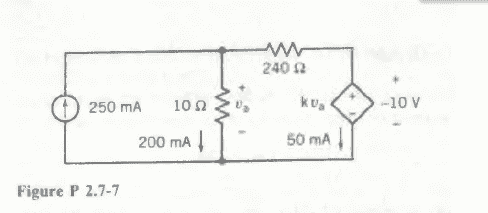# Dependent sources - can the gain (g) be negative?

lillybeansSo the goal in this case is to find k, the gain of the dependent source shown as a diamond.

Formula for dependent voltage sources:
V=g*V0

In this case
V=k*Va

I would get a negative value for k, because k=V/Va=-10V/(0.2A*10Ω)=-5

But can you have a negative value for k? Is that possible? Or did I get the signs wrong?

Last edited: